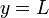# Horizontal asymptote

## Definition

### For a curve

Fill this in later

### For a function

Suppose$f$ is a function whose domain contains an interval of the form$(a,\infty)$ for some real number$a$ or an interval of the form$(-\infty,b)$ for some real number$b$. We say that the line$y = L$ is a horizontal asymptote for the graph of$f$ if one or both of these holds:$\! \lim_{x \to \infty} f(x) = L, \qquad \lim_{x \to -\infty} f(x) = L$